## 24 Feb JavaScript Operators

Operators perform operations by taking one or more values, to give another value. These operations are performed on variables and values. Operators in JavaScript are discussed here. For example:

The following are the operators in JavaScript:

• Arithmetic Operators
• Assignment Operators
• Comparison Operators
• Logical Operators
• Conditional Operators

Let us understand the operators one by one:

## JavaScript Arithmetic Operators

Arithmetic operators in JavaScript perform arithmetical operations, such as addition, subtraction, division, multiplication, etc.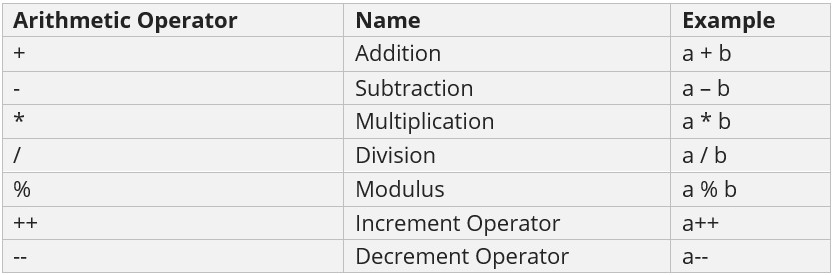Let us see an example to understand the Arithmetic Operators in JavaScript:

Output## JavaScript Assignment Operators

Use the Assignment Operator when you need to assign values to a variable. It also includes the Arithmetic Assignment Operators: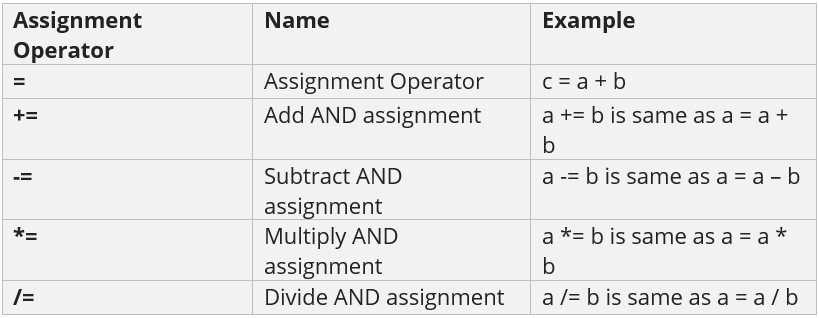Let us see an example to understand the Assignment Operator in JavaScript:

Output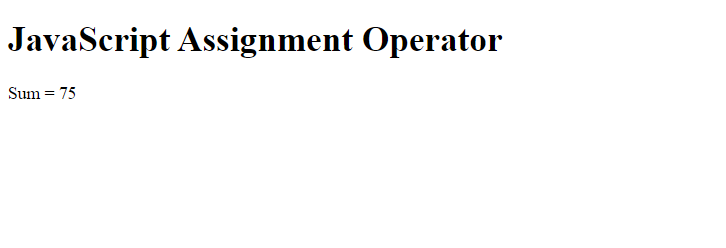## JavaScript Relational/ Comparison Operators

Compare two values with JavaScript relational operators, which are also known as Comparison Operators. Let us say a = 3, b =5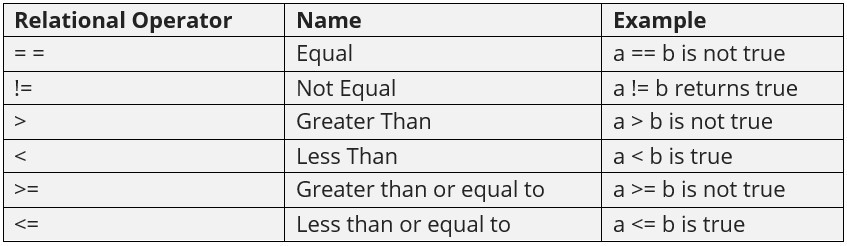Let us see an example to understand the Relational Operator in JavaScript:

Output## JavaScript Logical Operators

Logical operators combine conditional statements. Considering Boolean variables, a and b. Let us see an example of Logical Operators in JavaScript: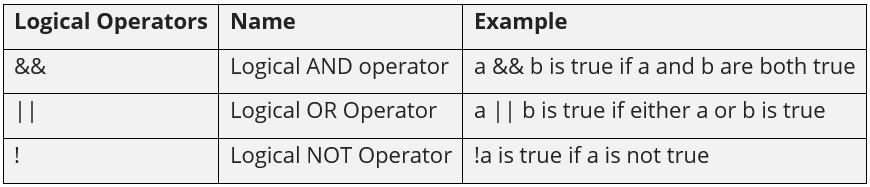Let us see an example to understand Logical Operators in JavaScript:

Output## JavaScript Conditional Operator

Conditional operator evaluates Boolean expressions, with three operands. It is also known as the ternary operator. The role is to assign a value to a variable, from two given set options. The following is the syntax:

As shown above, if the condition is true, value 1 will get displayed, else value 2.

Let us see an example to implement the Conditional Operator in JavaScript:

Output[email protected]

We work to create programming tutorials for all.